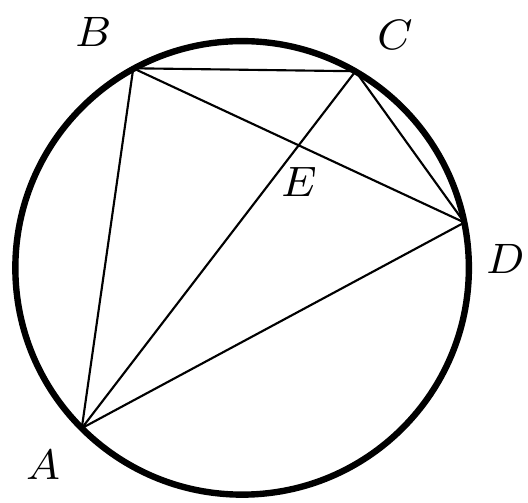###### back to index | new

Let quadrilateral $ABCD$ inscribe a circle. If $BE=ED$, prove $$AB^2+BC^2 +CD^2 + DA^2 = 2AC^2$$Let $ABCD$ be a square. Point $E$ is outside $ABCD$ such that $DE=CD$ and $\angle{AED}=15^\circ$. Show $\triangle{DCE}$ is equilateral.

Four sides of a concyclic quadrilateral have lengths of 25, 39, 52, and 60, in that order. Find the circumference of its circumcircle.